# Resources tagged with: GeoGebra

Filter by: Content type:
Age range:
Challenge level:

### There are 90 results

Broad Topics > Physical and Digital Manipulatives > GeoGebra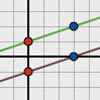### Parallel Lines

##### Age 11 to 14 Challenge Level:

How does the position of the line affect the equation of the line? What can you say about the equations of parallel lines?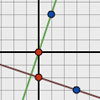### Perpendicular Lines

##### Age 14 to 16 Challenge Level:

Position the lines so that they are perpendicular to each other. What can you say about the equations of perpendicular lines?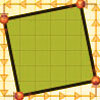### At Right Angles

##### Age 14 to 16 Challenge Level:

Can you decide whether two lines are perpendicular or not? Can you do this without drawing them?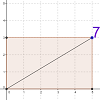### Exploring Diagonals

##### Age 11 to 16

Move the corner of the rectangle. Can you work out what the purple number represents?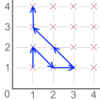### Coordinates of Corners

##### Age 11 to 16

Use the applet to make some squares. What patterns do you notice in the coordinates?### Areas from Vectors

##### Age 11 to 16

Use the applet to explore the area of a parallelogram and how it relates to vectors.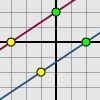### Translating Lines

##### Age 11 to 14 Challenge Level:

Investigate what happens to the equation of different lines when you translate them. Try to predict what will happen. Explain your findings.### Squirty

##### Age 14 to 16 Challenge Level:

Using a ruler, pencil and compasses only, it is possible to construct a square inside any triangle so that all four vertices touch the sides of the triangle.### Dice/spinner Interactives

##### Age 11 to 14 Challenge Level: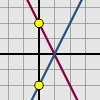### Reflecting Lines

##### Age 11 to 14 Challenge Level:

Investigate what happens to the equations of different lines when you reflect them in one of the axes. Try to predict what will happen. Explain your findings.### Solving Together - Estimating Angles

##### Age 11 to 14

Week 2
How well can you estimate angles? Playing this game could improve your skills.### Impossible Picture?

##### Age 14 to 16 Challenge Level:

Under what circumstances can you rearrange a big square to make three smaller squares?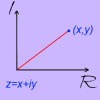### A Brief Introduction to the Argand Diagram

##### Age 14 to 18 Challenge Level:

Complex numbers can be represented graphically using an Argand diagram. This problem explains more...##### Age 14 to 16 Challenge Level:

Kyle and his teacher disagree about his test score - who is right?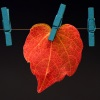### Number Lines in Disguise

##### Age 7 to 14 Challenge Level:

Some of the numbers have fallen off Becky's number line. Can you figure out what they were?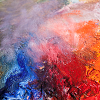### Mixing More Paints

##### Age 14 to 16 Challenge Level:

Can you find an efficent way to mix paints in any ratio?### L-triominoes

##### Age 14 to 16 Challenge Level:

L triominoes can fit together to make larger versions of themselves. Is every size possible to make in this way?### Sine and Cosine for Connected Angles

##### Age 14 to 16 Challenge Level:

The length AM can be calculated using trigonometry in two different ways. Create this pair of equivalent calculations for different peg boards, notice a general result, and account for it.### Mixing Paints

##### Age 11 to 14 Challenge Level:

Can you work out how to produce different shades of pink paint?### Overlap

##### Age 11 to 14 Challenge Level:

A red square and a blue square overlap so that the corner of the red square rests on the centre of the blue square. Show that, whatever the orientation of the red square, it covers a quarter of the. . . .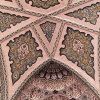### Angles Inside

##### Age 11 to 14 Challenge Level:

Draw some angles inside a rectangle. What do you notice? Can you prove it?### Beelines

##### Age 14 to 16 Challenge Level:

Is there a relationship between the coordinates of the endpoints of a line and the number of grid squares it crosses?### The Farmers' Field Boundary

##### Age 11 to 14 Challenge Level:

The farmers want to redraw their field boundary but keep the area the same. Can you advise them?### The Medieval Octagon

##### Age 14 to 16 Challenge Level:

Medieval stonemasons used a method to construct octagons using ruler and compasses... Is the octagon regular? Proof please.### Points in Pairs

##### Age 14 to 16 Challenge Level:

Move the point P to see how P' moves. Then use your insights to calculate a missing length.### Triangle in a Triangle

##### Age 14 to 16 Challenge Level:

Can you work out the fraction of the original triangle that is covered by the inner triangle?### Where Is the Dot?

##### Age 14 to 16 Challenge Level:

A dot starts at the point (1,0) and turns anticlockwise. Can you estimate the height of the dot after it has turned through 45 degrees? Can you calculate its height?##### Age 14 to 16 Challenge Level:### Nine Colours

##### Age 11 to 16 Challenge Level:

Can you use small coloured cubes to make a 3 by 3 by 3 cube so that each face of the bigger cube contains one of each colour?### Strolling Along

##### Age 14 to 18 Challenge Level:

What happens when we multiply a complex number by a real or an imaginary number?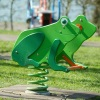### Surprising Equalities

##### Age 14 to 18 Challenge Level:

Take any triangle, and construct squares on each of its sides. What do you notice about the areas of the new triangles formed?### Mapping the Territory

##### Age 14 to 18 Challenge Level:

Can you devise a system for making sense of complex multiplication?### Opening the Door

##### Age 14 to 18 Challenge Level:

What happens when we add together two complex numbers?### Sine and Cosine

##### Age 14 to 16 Challenge Level:

The sine of an angle is equal to the cosine of its complement. Can you explain why and does this rule extend beyond angles of 90 degrees?### Pythagoras Proofs

##### Age 14 to 16 Challenge Level:

Can you make sense of these three proofs of Pythagoras' Theorem?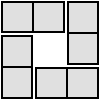### 4 Dom

##### Age 5 to 16 Challenge Level:

Use these four dominoes to make a square that has the same number of dots on each side.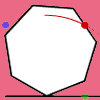### Up and Across

##### Age 11 to 14 Challenge Level:

Experiment with the interactivity of "rolling" regular polygons, and explore how the different positions of the dot affects its vertical and horizontal movement at each stage.### Round and Round a Circle

##### Age 14 to 16 Challenge Level:

Can you explain what is happening and account for the values being displayed?### Polar Coordinates

##### Age 14 to 18

Cartesian Coordinates are not the only way!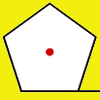### How Far Does it Move?

##### Age 11 to 14 Challenge Level:

Experiment with the interactivity of "rolling" regular polygons, and explore how the different positions of the red dot affects the distance it travels at each stage.### Spinners Environment

##### Age 5 to 18 Challenge Level:

A tool for generating random integers.### Vanishing Point

##### Age 14 to 18 Challenge Level:

How can visual patterns be used to prove sums of series?##### Age 14 to 16 Challenge Level:

The points P, Q, R and S are the midpoints of the edges of a non-convex quadrilateral.What do you notice about the quadrilateral PQRS and its area?### Same Length

##### Age 11 to 16 Challenge Level:

Construct two equilateral triangles on a straight line. There are two lengths that look the same - can you prove it?### Into the Wilderness

##### Age 14 to 18 Challenge Level:

Let's go further and see what happens when we multiply two complex numbers together!### Subtended Angles

##### Age 11 to 14 Challenge Level:

What is the relationship between the angle at the centre and the angles at the circumference, for angles which stand on the same arc? Can you prove it?### Rolling Around

##### Age 11 to 14 Challenge Level:

A circle rolls around the outside edge of a square so that its circumference always touches the edge of the square. Can you describe the locus of the centre of the circle?### Right Angles

##### Age 11 to 14 Challenge Level:

Can you make a right-angled triangle on this peg-board by joining up three points round the edge?### Squaring the Circle and Circling the Square

##### Age 14 to 16 Challenge Level:

If you continue the pattern, can you predict what each of the following areas will be? Try to explain your prediction.### Triangles in Circles

##### Age 11 to 14 Challenge Level:

Can you find triangles on a 9-point circle? Can you work out their angles?